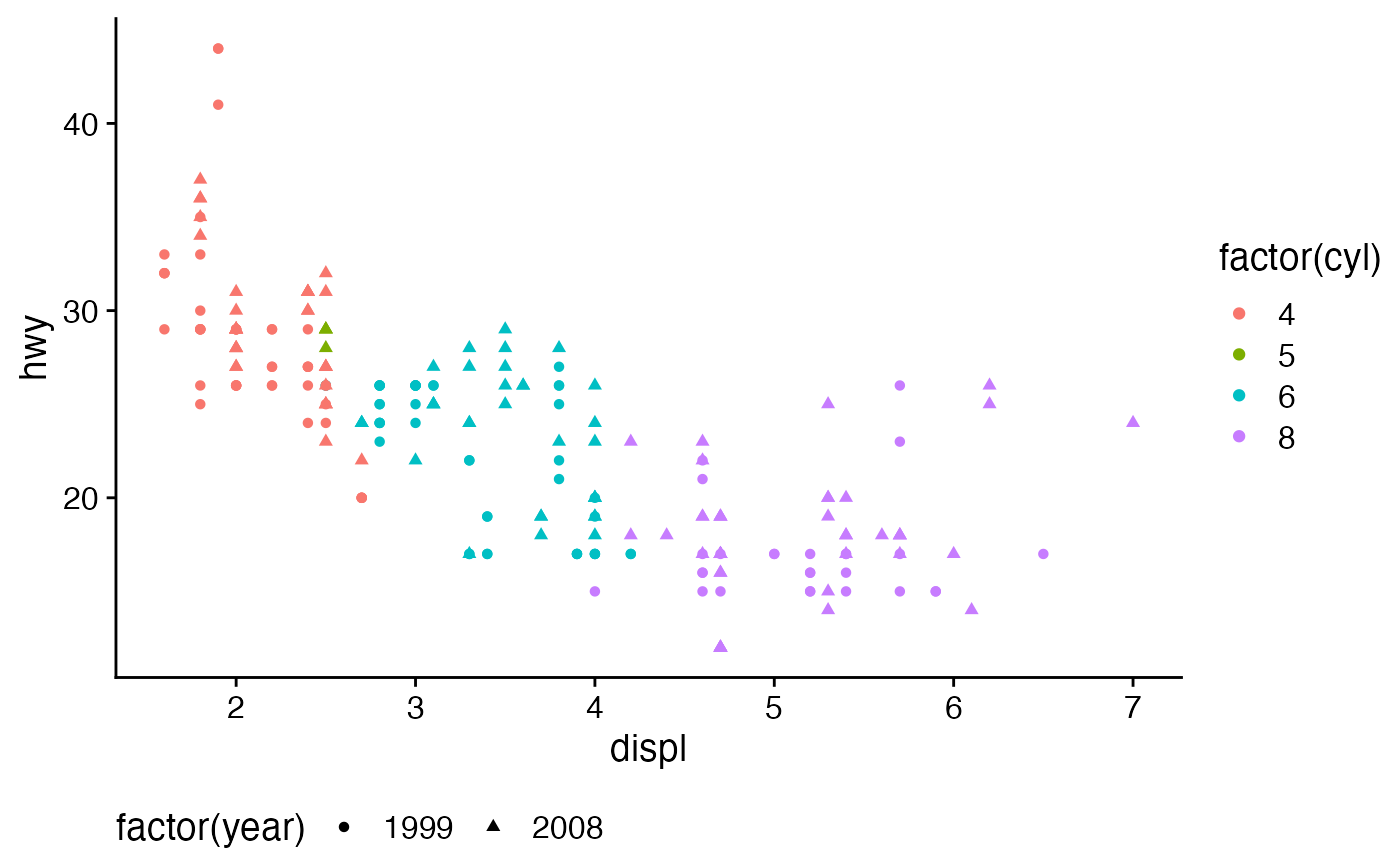This function extracts just the legend from a ggplot

get_legend(plot)

## Arguments

plot A ggplot or gtable from which to retrieve the legend

## Value

A gtable object holding just the legend or NULL if there is no legend.

## Examples

library(ggplot2)
theme_set(theme_half_open())

p1 <- ggplot(mtcars, aes(mpg, disp)) + geom_line()
plot.mpg <- ggplot(mpg, aes(x = cty, y = hwy, colour = factor(cyl))) + geom_point(size=2.5)
# Note that these cannot be aligned vertically due to the legend in the plot.mpg
ggdraw(plot_grid(p1, plot.mpg, ncol=1, align='v'))
#> Warning: Graphs cannot be vertically aligned unless the axis parameter is set. Placing graphs unaligned.legend <- get_legend(plot.mpg)
plot.mpg <- plot.mpg + theme(legend.position='none')
# Now plots are aligned vertically with the legend to the right
ggdraw(plot_grid(plot_grid(p1, plot.mpg, ncol=1, align='v'),
plot_grid(NULL, legend, ncol=1),
rel_widths=c(1, 0.2)))